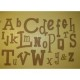Posted by: Prof. Kate | September 23, 2008

## What is a theorem and a proof?

The substance of formal mathematics is definition, theorem and proof.

A theorem is simply a mathematical statement of something which is true, for example “16 is a perfect square.”  In order to understand a mathematical statement, we must understand the relevant definitions.  In this case, we need to know what it means to be a perfect square.  If we want to be formal about it, we can state it as a defintion:

Definition. A perfect square is any natural number which is the square of another natural number.

To understand this definition, we need to know the definition of a natural number, too.  Often one has to trace back definitions in this way.  But I think we know what natural numbers are: 1, 2, 3, 4, 5, 6, 7, 8, etc.

Now we can state our theorem:

Theorem. 16 is a perfect square.

And, ideally, we would like to prove it.  A proof is just a logical argument that shows the statement is true.  In this case, it’s fairly short.

Proof. We know that 4 is a natural number.  We can calculate$4^2 = 16$.  Therefore 16 is the square of a natural number.$\square$

The argument should consist of calling on previous knowledge, or other theorems we have already proven, and definitions, and deductions based on these.  Ideally it includes lots of details, so others reading it can be completely convinced.

There are, of course, lots of mathematical statements that aren’t theorems, like “13 is a perfect square.”  This is a perfectly well-defined statement, it just isn’t true.

That’s what mathematicians do.  We make mathematical statements, we try to figure out if they are true, and if they are, prove them.  If they aren’t, we try to change them to make them true, and then prove them.  Of course, we don’t pick random statements like the one above (it really isn’t that interesting).  Think of mathematicians as artists working in a strict and difficult form, like fugue or sonnet form.  We try to find beautiful mathematical structures, and describe them in the formal manner of definition, theorem, proof.

## Responses

1.Thanks for sharing this can get very confusing.